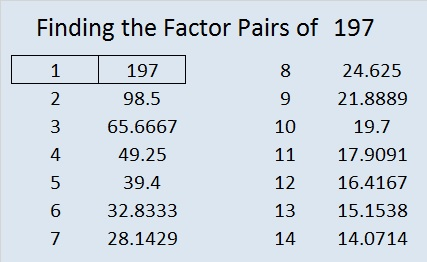# 197 and Level 5

• 197 is a prime number.
• Prime factorization: 197 is prime.
• The exponent of prime number 197 is 1. Adding 1 to that exponent we get (1 + 1) = 2. Therefore 197 has exactly 2 factors.
• Factors of 197: 1, 197
• Factor pairs: 197 = 1 x 197
• 197 has no square factors that allow its square root to be simplified. √197 ≈ 14.0356688How do we know that 197 is a prime number? If 197 were not a prime number, then it would be divisible by at least one prime number less than or equal to √197 ≈ 14. Since 197 cannot be divided evenly by 2, 3, 5, 7, 11, or 13, we know that 197 is a prime number.Excel file of puzzles and previous week’s factor solutions: 10 Factors 2014-08-04